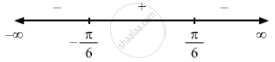Share

F(X) = Sin 2x − X, − π 2 < < X < π 2 . - CBSE (Arts) Class 12 - Mathematics

Question

f(x)=sin2x-x, -pi/2<=x<=pi/2

Solution

$\text { Given }: \hspace{0.167em} f\left( x \right) = \sin 2x - x$

$\Rightarrow f'\left( x \right) = 2 \cos 2x - 1$

$\text { For a local maximum or a local minimum, we must have }$

$f'\left( x \right) = 0$

$\Rightarrow 2 \cos 2x - 1 = 0$

$\Rightarrow \cos 2x = \frac{1}{2}$

$\Rightarrow x = \frac{- \pi}{6} or \frac{\pi}{6}$Since  f'(x) changes from positive to negative when x increases through $\frac{\pi}{6}$ x = $\frac{\pi}{6}$ is the point of local maxima.

The local maximum value of  f (x) at x = $\frac{\pi}{6}$ is given by $\sin \left( \frac{\pi}{3} \right) - \frac{\pi}{6} = \frac{\sqrt{3}}{2} - \frac{\pi}{6}$

Sincef '(x) changes from negative to positive when x increases through $- \frac{\pi}{6}$ x = $- \frac{\pi}{6}$  is the point of local minima.
The local minimum value of  f (x)  at x = $- \frac{\pi}{6}$ is given by $\sin \left( \frac{- \pi}{3} \right) + \frac{\pi}{6} = \frac{\pi}{6} - \frac{\sqrt{3}}{2}$
Is there an error in this question or solution?

Video TutorialsVIEW ALL 

Solution F(X) = Sin 2x − X, − π 2 < < X < π 2 . Concept: Graph of Maxima and Minima.
S